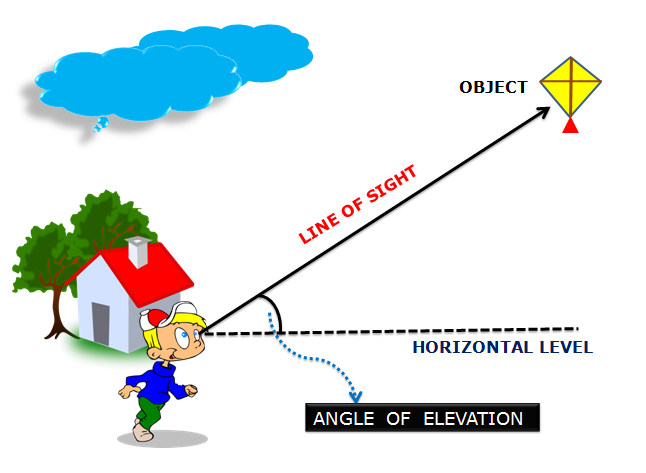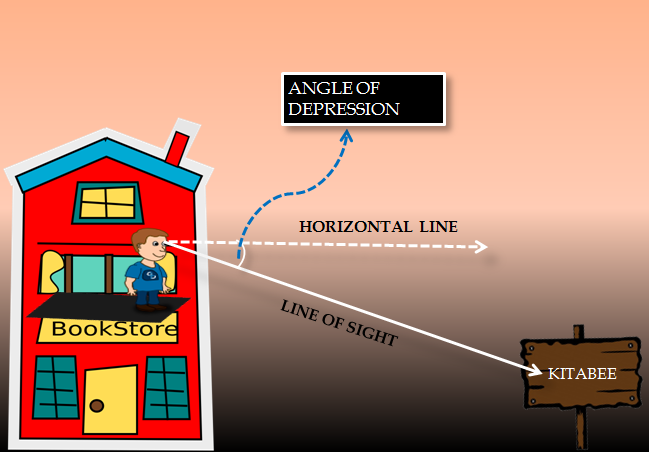# Trigonometry

#### Heights And Distances - 1

Heights And Distances - 1

Heights And Distances

The chapter on Heights and Distances involves the application of Trigonometric Ratios in solving problems related to the calculation of heights and distances of various objects without actually measuring them. It finds its place in Astronomy and Geography where the distances between celestial bodies can be calculated and also heights of various mountain peaks can be determined by using simple trigonometric ratios. But, before we start solving problems, let’s discuss some basic concepts and terms that we shall encounter while studying this chapter.

Angle of Elevation:Angle of Depression:W

With the help of a few solved examples you will understand how to make use of trigonometric ratios to the find the height of objects or the distance between the objects involved in the questions.